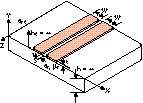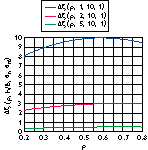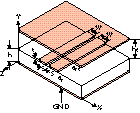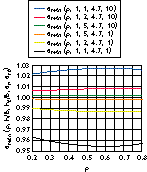AN ANALYSIS OF ENCLOSED COPLANAR STRIPS

### Abstract:

With the increasing use of high speed digital circuits in modern electronic devices, coplanar stripline (CPS) transmission lines are of major importance as interconnecting lines. For example, the high speed digital circuits used in differential receivers operate best when fed using CPS balanced transmission lines. However, the theoretical CPS geometry and associated current design equations do not take into account the presence of a bottom ground conductor, which is often present in printed circuit board MIC or MMIC configurations. When a bottom ground conductor is inserted below a CPS, the resulting structure is a conductor-backed coplanar strip (CBCPS). Such a configuration is often encountered in microwave mixers as well. In addition, until today no simulation model in CAD tools existed for these CBCPS transmission lines. Using current quasistatic analysis, this article develops simple closed-form equations for characteristic impedance and effective dielectric constant for CBCPSs and considers the effect of a top ground cover. These equations are well suited for implementation in CAD simulations tools.

TELIT SpA, Satellite Division

Rome, Italy

The original CPS geometry is shown in Figure 1. In practice, two conductor strips of equal width w are placed near each other at a distance s, positioned above a dielectric plane of theoretically infinite thickness h. This transmission line was first introduced by C.P. Wen1 as the complementary case of another well-known and used transmission line, the coplanar waveguide (CPW), shown in Figure 2. Wen used a conformal mapping technique to obtain the characteristic impedance z and effective relative dielectric constant ere for CPW. He made the assumptions of a dielectric of infinite thickness and conductors of negligible thickness t. By duality, the results of this CPW analysis are applied to CPS.Fig. 1 The original CPS geometry.

The first practical difference from the original CPS analysis is that, in practice, the substrate's thickness h is a finite dimension. The first study to account for this change was made by J.B. Knorr and K.D. Kuchler2 with a full-wave analysis, and then by others with quasistatic3,4 or full-wave methods.5 Assuming that complementary lines give equal phase speed for a signal, I.J. Bahl et al.6 have developed expressions for CPS characteristic impedance and effective dielectric constant that consider the finite h value.Fig. 2 Coplanar waveguide.

The second difference is the inevitable conductor thickness t. The resulting effect was originally evaluated by H.A. Wheeler7 for the microstrip case and subsequently applied to CPS by K.C. Gupta et al. In detail, an extra width dw function of t is added to each strip width, and a new CPS with zero-thickness conductors is evaluated. The dimensions of the CPS are

whereUnequal-width CPS configurations have been studied by I. Kneppo and J. Gotzman8 and losses have been examined by K.C. Gupta6 et al. High speed pulse propagation in CPSs has also been investigated,9,10 verifying the good capability of this transmission line for that purpose.

CBCPS ANALYSIS

The CBCPS geometrical structure to be analyzed is shown in Figure 3. It is assumed that a pure TEM mode propagates in this transmission line. (This assumption is true only if a homogeneous dielectric media surrounds the strips.) The conductor thickness t is assumed to be infinitesimal. The specialized theoretical analysis of this structure is performed once for the top-side half-space and once for the bottom dielectric space. This method of proceeding is suitable when a conformal transformation method11 is employed for the analysis, as in this case.

Observing the electric field strength lines shown in Figure 4, an ideal electric plane (IEP) can be placed at the middle of the spacing s and orthogonal to the ground plane to simplify the analysis. According to the TEM propagation mode this assumption is believed to be true. Due to this symmetry, only one-half of the bottom side, indicated with BL and BR, is analyzed, as shown in Figure 5. This geometry will be transformed into the T-plane structure displayed in the figure. However, note that the capacitance evaluated in the T-plane structure is two times that for the complete CBCPS structure since one-half of the distance s is being considered in the T plane.Fig. 3 The CBCPS's geometrical structure.Fig. 4 Electric field strength.Fig. 5 Analysis of one-half of the structure.Fig. 6 Ct evaluation.This transformation maps the BR region of the Z plane into the upper plane of the R plane. Solving the previous simple integral and applying the t6 = 0´r6 = -1 and z1 = -jh´r1 = 0 relationships, the desired transformation isandThe geometry in the R plane is then mapped to the T plane through another Schwarz-Christoffel12 transformation t = CI2 + D. The integral involved in such a transformation is expressed asthat with the variable substitution

can be transformed in the elliptic integral of first kind F(v, pb),13 with parameter pb given byThus, the final transforming equation can be written as

where Q and E are constants.

Applying the relationships t6 = 0´r6 = -1, t4 = -1 - jd´r = r4 and t5 = -jd´r = r5, the desired value of d is K(pb)/K(pb'), where K() is the complete elliptic integral of the first kind.13 The primed parameters are the so-called complementary parameters and are evaluated as p' = (1 - p2)0.5. Therefore, the resulting capacitance per unit length of the T-plane structure is Cbs = e0erK(pb')/K(pb) and, consequently, the bottom capacitance of the Z plane isThe ratio of the elliptic integral was evaluated by W. Hilberg,14 and is given byTo evaluate the total capacitance C per unit length of the CBCPS structure the top capacitance Ct of the unlimited top side of the CPS must be evaluated, as shown in Figure 6. The Schwarz-Christoffel transformation t = GI3 + H involves the integral I3, which is given bythat with the variable substitution

z = z4q

can be rewritten aswhereThe final transforming equation is

where L and M are constants.

Such a transformation maps the y > 0 half plane of the Z plane into the internal region of the rectangle in the T plane. Then, applying the relationships t2 = -1 + je´z = z2, t3 = -1´z = z3 and t7 = 0´z = z7, the desired value of e is K(p')/K(p), and the resulting capacitance per unit length Ct for the top side of the Z plane iswhere ert is the relative dielectric constant of the dielectric above the substrate. ert = 1 is assumed in every function unless otherwise stated. Therefore, the resulting total capacitance per unit length C of the CBCPS isThen, using the previous equation in the general definition of the effective relative dielectric constant for the quasistatic case yieldsEvaluating the effective relative dielectric constant for the original CPS producesThe characteristic impedance z can be obtained by the well-known equation for TEM transmission lines. z = 1/Cv, where v is the light propagation in the medium. The final expression isSince, after a simple De l'Hopital application,the elliptic integrals evaluated with pb or p have the same value for hÆ and, consequently,In addition, it is simple to demonstrate thatand, consequently, using Hilberg's equation,andEquation 23 is physical since when h = 0 the two conductors are short circuited and the charactersitic impedance of the line is, of course, zero while Equation 24 is nonphysical. In fact, if h = 0, no substrate with a er value exists, and the solution to Equation 24 is only analytical, showing that when h = 0 the electromagnetic field tends to be completely concentrated in the substrate. However, it has no physical result since for h = 0 no transmission line exists and z = 0.

The value eren (p, h/b, er, ert) of ereh normalized to ere is shown in Figure 7 as a function of p for er = 4.7. Two sets of three curves each are displayed: one set is for ert = 1; the other has ert = 10. Three curves are plotted for each set in accordance with values of h/b = 1, 2, 5. Note that when h increases, the value of eren decreases toward 1 if er > ert, or increases toward 1 if er < ert. It can be observed that the bottom ground plane forces the electromagnetic field to be concentrated in the bottom substrate. Since the maxima or minima of the curves correspond to p ª 0.6, the electromagnetic field for w/s ª 0.6 is maximally concentrated in the substrate. An interesting graph showing that erenÆ1 for hÆ is shown in Figure 8 for w = 200 mm, s = 100 mm and er = 10 and 2.2 vs. h in micrometers.Fig. 7 eren (p, h/b, er, ert) as a function of p.Fig. 8 eren vs. h.Fig. 9 Characteristic impedance vs. p.

Figure 9 shows the characteristic impedance for the CBCPS for er = 10 as a function of p and h/b equal to 0.5, 1 and 2. zu is the impedance of the unlimited CPS (that is, the original CPS), given byAn interesting function is the percentage error Dz between z and zu vs. p, defined asand shown in Figure 10. Note that since h/b = 3 the error is below three percent, and this characteristic is quite independent of the er value.Fig. 10 Percentage error between the CPSG characteristic impedance and the impedance of the original CPS.Fig. 11 fm vs. p for various values of s.

It is important to observe that small values of p mean small values for s and/or large values for w. However, this last condition is not advisable in practice since a large w value causes a possible growth of spurious modes. The existance of these unwanted modes in CBCPS can be rigorously justified with full-wave methods15 and is still under investigation.16 Higher order modes, essentially transverse modes, are also possible starting at a frequency fm approximated bywhere

vo = free space speed of light

Figure 11 shows the results when the expression in Equation 17 is substituted for ereh. Using different values of h/b it is simple to verify that the frequency fm decreases if h/b is decreased.

CONDUCTOR-BACKED AND TOP-SHIELDED CPS ANALYSIS

Figure 12 shows the geometrical structure of a conductor-backed and top-shielded CPS. In practice, a top ground conductor is placed over the CPS's two conductor layers at a distance ht. The analysis of this structure is performed in a fashion similar to the previously completed evaluation of the bottom capacitance. However, in this case the analysis also must be applied for the region between the top ground conductor and the CPS layer. Therefore, the equivalent capacitance Cs of the structure and its effective relative dielectric constant are expressed asFig. 12 A conductor-backed and top-shielded coplanar strip.Fig. 13 eretn vs p.where pt is given by the previously displayed graph of eren vs. h, replacing h with ht. Note also in this case thatThe characteristic impedance is now given bywhere eret is given in Equation 29. Of course, the primed parameters pb and pt are the complementary parameters of pb and pt.Fig. 14 Change in characteristic impedance vs. p.

The value eretn (p, h/b, ht/b, er, ert) of eret normalized to ereh is shown in Figure 13 as a function of p with h/b = 1 and er = 4.7. Two sets of three curves each are displayed: one set is for ert = 1; the other has ert = 10. For each set, three curves are drawn in accordance with ht/b = 1, 2, 5 values. Note that when ht increases the value of eretn decreases toward 1 if er < ert, or increases toward 1 if er > ert. It is simple to verify thatThe percentage error Dzt between zt and z vs. p, defined asis shown in Figure 14, with h/b = ht/b = 1.

CONCLUSION

A quasistatic approach has been used to study CBCPS. Formulas for characteristic impedance and effective dielectric constants have been given, which are well suited to be simply implemented in CAD simulation tools to help design microwave devices employing balanced transmission lines. The results of the analysis show that the effect of the bottom ground conductor decreases the characteristic impedance of the CPS. A top-shielded CBCPS also has been studied, and analysis formulas have been given for this transmission line configuration.

References

1. C.P. Wen, "Coplanar Waveguide: A Surface Strip Transmission Line for Nonreciprocal Gyromagnetic Device Application," IEEE Transactions on Microwave Theory and Techniques, Dec. 1969, pp. 1087-1090.

2. J.B. Knorr and K.D. Kuchler, "Analysis of Coupled Slots and Coplanar Strips on Dielectric Substrates," IEEE Transactions on Microwave Theory and Techniques, July 1975, pp. 541-548.

3. V.F. Hanna, "Finite Boundary Corrections to Coplanar Stripline Analysis," Electronic Letters, July 1980, pp. 604-606.

4. G. Ghione and C. Naldi, "Analytical Formulas for Coplanar Lines in Hybrid and Monolithic MICs," Electronic Letters, Feb. 1984, pp. 179-181.

5. S.G. Pintzos, "Full Wave Spectral Domain Analysis of Coplanar Strips," IEEE Transactions on Microwave Theory and Techniques, Feb. 1991, pp. 239-246.

6. K.C. Gupta, R. Garg and I.J. Bahl, Microstrip Lines and Slotlines, Artech House, Norwood, MA, 1979, p. 276.

7. H.A. Wheeler, "Transmission Line Properties of a Strip on a Dielectric Sheet on a Plane," IEEE Transactions on Microwave Theory and Techniques, Aug. 1977, p. 718.

8. I. Kneppo and J. Gotzman, "Basic Parameters of Nonsymmetrical Coplanar Lines," IEEE Transactions on Microwave Theory and Techniques, Aug. 1977, p. 718.

9. M.Y. Frankel, R.H. Voelker and J.N. Hilfiker, "Coplanar Transmission Lines on Thin Substrates for High Speed Low Loss Propagation," IEEE Transactions on MIT, Mar. 1994, pp. 396-402.

10. H. Cheng, J.F. Whitaker, T.M. Weller and L.P.B. Katehi, "Terahertz Bandwidth Pulse Propagation on a Coplanar Stripline Fabricated on a Thin Membrane," MGWL, Mar. 1994, pp. 89-91.

11. F. Di Paolo, Networks and Devices Using Planar Transmission Lines, CRC Press, 2000 (ISBN 0-8493-1835-1).

12. S. Ramo, J.R. Whinnery and T. Van Duzer, Fields and Waves in Communication Electronics, J. Wiley & Sons, 1965, p. 189.

13. M. Abramowitz and I.A. Stegun, Handbook of Mathematical Functions, Dover Editions, 1970, p. 589.

14. W. Hilberg, "From Approximations to Exact Relations for Characteristic Impedances," IEEE Transactions on Microwave Theory and Techniques, May 1969, pp. 259-265.

15. M. Tsuji, H. Shigesawa and A.A. Oliner, "Simultaneous Propagation of Both and Leaky Dominant Modes on Conductor Backed Coplanar Strips," IEEE MTT Symposium Digest, 1993, pp. 1295-1298.

16. A.B. Yakovlev and G.W. Hanson, "On the Nature of Critical Points in Leakage Regimes of Conductor Backed Coplanar Strip Line," IEEE Transactions on Microwave Theory and Techniques, Jan. 1997, pp. 87-94.

Franco Di Paolo received his doctorate degree in electronic engineering in 1984 from Università degli studi di Roma 'La Sapienza.' He started working at Ericsson, Rome in 1986 as an RF hardware designer and joined Elettronica SpA, Rome in 1992 as a microwave and RF hardware designer in wide bandwidth microwave circuits and MMICs. Currently, he is chief research engineer at TELIT SpA's Satellite Division in Rome. Di Paolo's research interests include applied electromagnetism and RF/microwave analog hardware design. He can be reached via e-mail at franco.di.paolo@ntt.it.